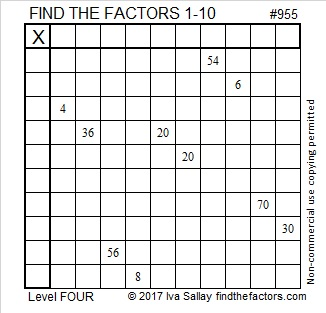# 955 and Level 4

This level 4 puzzle has ten clues and two of them are the same number! No worries! There is still only one way to write the numbers from 1 to 10 in the first column and the top row so that this puzzle will function like a multiplication table. Can you figure out where to place those numbers?Print the puzzles or type the solution in this excel file: 10-factors-951-958

Here’s some information about the number 955:

It is the hypotenuse of a Pythagorean triple:
573-764-955 which is (3-4-5) times 191

It is a palindrome in two other bases:
32323 in BASE 4 because 3(4⁴) + 2(4³) + 3(4²) + 2(4¹) + 3(4⁰) = 955
353 BASE 17 because 3(17²) + 5(17¹) + 3(17⁰) = 955

• 955 is a composite number.
• Prime factorization: 955 = 5 × 191
• The exponents in the prime factorization are 1 and 1. Adding one to each and multiplying we get (1 + 1)(1 + 1) = 2 × 2 = 4. Therefore 955 has exactly 4 factors.
• Factors of 955: 1, 5, 191, 955
• Factor pairs: 955 = 1 × 955 or 5 × 191
• 955 has no square factors that allow its square root to be simplified. √955 ≈ 30.90307This site uses Akismet to reduce spam. Learn how your comment data is processed.